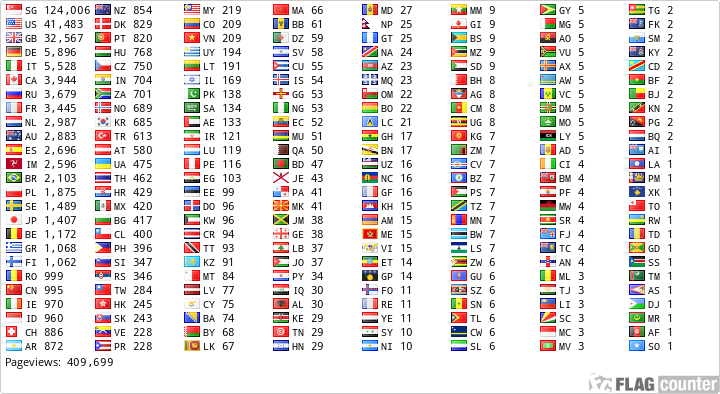# IP Math’s

986 viewsGiven the IP address of 142.168.45.79 / 255.255.255.240 is the IP address of 142.168.45.79 a valid address for a user on that network?

So what are we really interested in? this is easy, taking the address shown above and the subnet given we can tell that we are only interested in the last octet, this is because all of the subnet octets are 255 except the last one, therefore that is the only one that we are interested in, and this is 240.

So we now need to work out what is available on this subnet, so we will write out a table similar to the one below.

 128 192 224 240 248 252 254 255 128 64 32 16 8 4 2 1

The easiest way to remember this is that you always start the first column always with 128, so in the first column place 128, i.e.

 128 128

Then concentrate on the second row only, for this just half the previous number, so that you end up with the following:

 128 192 128 64 32 16 8 4 2 1

Now to get the top row, just add 128 + 64 = 192.

 128 192 224 128 64 32 16 8 4 2 1

then the next column will be 192 + 32 = 224, and so on, till you get the following table.

 128 192 224 240 248 252 254 255 128 64 32 16 8 4 2 1

Now we need to convert the Network address of 142.168.45.79 to binary, as above we know that we are only interested in the last octet so all we need to worry about here is the .79 part of the IP address.

So drawing a line under the above table, and only taking into account the second row of numbers, put a ‘1’ into the columns that you need to make up ’79’, for the .79 portion of the IP address, and ‘0’ in the columns that are not required.

This should look like the following table.

 128 192 224 240 248 252 254 255 The Subnet 128 64 32 16 8 4 2 1 The Network ID 0 1 0 0 1 1 1 1 The Network ID

Now create a new row after this for the Subnet address, again we are only interested in the last octet which is ‘240’. For this tough you will need to place a ‘1’ in the column for the 240 and all the fields to the left of this, this will then look like the following table.

 128 192 224 240 248 252 254 255 The Subnet 128 64 32 16 8 4 2 1 The Network ID 0 1 0 0 1 1 1 1 The Network ID 1 1 1 1 0 0 0 0 The Subnet

Now you need to draw a line under this and using binary maths, which is very simple, we need to work out the ‘Network Address’, this address is not useable by users on the network, but lets us know what the address range is. For example a standard Class C address of 192.168.0.0 / 24 (IP address 192.168.0.0, Subnet Mask 255.255.255.0) would be explained as:

So now for the math’s bit. all that you need to remember is the following table.

 0 + 0 = 0 0 + 1 = 0 1 + 0 = 0 1 + 1 = 1

So taking this into account you should now have a table that looks like this.

 128 192 224 240 248 252 254 255 The Subnet 128 64 32 16 8 4 2 1 The Network ID 0 1 0 0 1 1 1 1 The Network ID 1 1 1 1 0 0 0 0 The Subnet 0 1 0 0 0 0 0 0 The Network Address

This tells us that the first network address is 64, or to be precise 142.168.45.64

Now we need to make the final calculation, this will give us the ‘Broadcast Address’, for this we will need to first add a new row under our last and call this ‘The Broadcast Address.

Now, we know that the IP address used is also on the subnet range of 255.55.255.240, and we know that we are only really interested in the last set of numbers, i.e. .240, and because of this we need to enter a ‘1’ in all of the boxes to the right of the 240 (The Subnet row), this should look like the following table.

 128 192 224 240 248 252 254 255 The Subnet 128 64 32 16 8 4 2 1 The Network ID 0 1 0 0 1 1 1 1 The Network ID 1 1 1 1 0 0 0 0 The Subnet 0 1 0 0 0 0 0 0 The Network Address 1 1 1 1 The Broadcast Address

Now to finish this, using the same math’s that we did earlier, look at the remaining four empty boxes at there respective columns, work this out, and this should look like the following table.

 128 192 224 240 248 252 254 255 The Subnet 128 64 32 16 8 4 2 1 The Network ID 0 1 0 0 1 1 1 1 The Network ID 1 1 1 1 0 0 0 0 The Subnet 0 1 0 0 0 0 0 0 The Network Address 1 1 1 1 The Broadcast Address

Finally we need to take these last two rows and convert them back to numerics thus.

 0 1 0 0 0 0 0 0 0.64 0 1 0 0 1 1 1 1 0.79

So, after all this, what do we know?

We now the following: